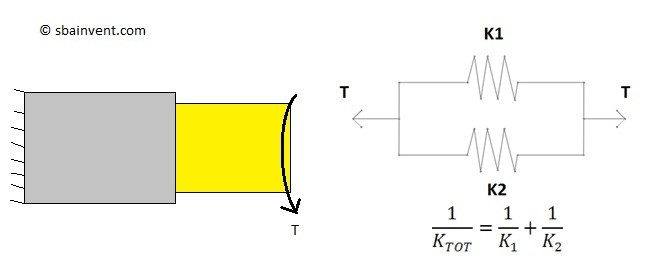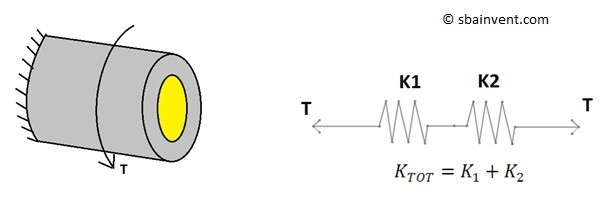# Torsional Stiffness

There are certain cases when calculated the torsional stiffness of a part might be useful. For one, in order calculate the natural frequencies of a part you need to determine the stiffness of the part. On the other hand if you want to determine the angle of twist of an object that is made out of dissimilar material for certain torque calculating the total stiffness can be useful. To calculate torsional stiffness the following equation would be used.

Eq 1  $k=\frac{T}{Φ}=\frac{JG}{L}$

$k$ = Stiffness

$T$ = Torque

$Φ$ = Angle of Twist

$J$ = Polar Moment of Inertia

$G$ = Shear Modulus

$L$ = Rod Length

This type of problem can be treated the same way you would treat resistors in an electrical circuit. There will be cases when the components can be considered in series, and there will be cases when the components can be considered in parallel.

Below is an example of two components in parallel.Below is an example of two components in series.